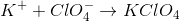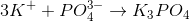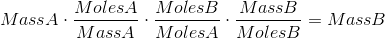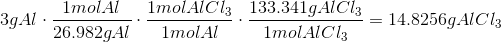# Homework 6

$$\newcommand{\vecs}{\overset { \rightharpoonup} {\mathbf{#1}} }$$ $$\newcommand{\vecd}{\overset{-\!-\!\rightharpoonup}{\vphantom{a}\smash {#1}}}$$$$\newcommand{\id}{\mathrm{id}}$$ $$\newcommand{\Span}{\mathrm{span}}$$ $$\newcommand{\kernel}{\mathrm{null}\,}$$ $$\newcommand{\range}{\mathrm{range}\,}$$ $$\newcommand{\RealPart}{\mathrm{Re}}$$ $$\newcommand{\ImaginaryPart}{\mathrm{Im}}$$ $$\newcommand{\Argument}{\mathrm{Arg}}$$ $$\newcommand{\norm}{\| #1 \|}$$ $$\newcommand{\inner}{\langle #1, #2 \rangle}$$ $$\newcommand{\Span}{\mathrm{span}}$$ $$\newcommand{\id}{\mathrm{id}}$$ $$\newcommand{\Span}{\mathrm{span}}$$ $$\newcommand{\kernel}{\mathrm{null}\,}$$ $$\newcommand{\range}{\mathrm{range}\,}$$ $$\newcommand{\RealPart}{\mathrm{Re}}$$ $$\newcommand{\ImaginaryPart}{\mathrm{Im}}$$ $$\newcommand{\Argument}{\mathrm{Arg}}$$ $$\newcommand{\norm}{\| #1 \|}$$ $$\newcommand{\inner}{\langle #1, #2 \rangle}$$ $$\newcommand{\Span}{\mathrm{span}}$$

5.49, 8.53

Chapter 5, #49

Write the formula for the compound that forms between Potassium and each of the polyatomic ions listed below:

a. Sulfate

b. Perchlorate

c. Nitrate

d. Phosphate

Strategy:

1. Identify the compound listed

2. Write out the chemical equation for each reaction

3. Balance the equations

Hints:

a. The formula for Sulfate is SO42-

b. The formula for Perchlorate is ClO4-

c. The formula for Nitrate is NO3-

d. The formula for Phosphate is PO43-

Solution:

a.b.c.d.Chapter 8, #53

For the reactions listed, calculate the theoretical yield for the products in grams using the listed initial amounts of the reactants.

a. 3g Al, 4g Cl

b. 9g Al, 18g Cl

c. 0.5g Al, 2g Cl

Strategy:

1. Find the molar mass of Al3+, Cl-, and AlCl3

2. Convert the initial amounts of the reactants in grams to moles of reactants (mass to mole conversion)

3. Convert the moles of reactants to moles of product (mole to mole conversion)

4. Convert the moles of product to mass of product in grams (mole to mass conversion)

Hints:

1. To convert from Mass A to Mass B to find grams of products, use the formula2. Theoretical yield - the amount predicted by a stoichometric calculation based on the number of moles of the reactants present to form the products

3. Keep in mind that the limiting reagent is whatever reactant gives you the smallest number because you can't create more than the limiting reagent can form, so the theoretical yield is the same as what the limiting reagent produces.

Solution:

a.The Theoretical Yield with 3g Al and 4g CL is 10.0295g AlCl3 with Cl being the limiting reagent.

b.The Theoretical Yield with 9g Al and 18g Cl is 44.4767g AlCl3 with Al being the limiting reagent.

c.The Theoretical Yield with0.5g Al and 2g Cl is2.4709g AlCl3 with Al being the limiting reagent.

Homework 6 is shared under a not declared license and was authored, remixed, and/or curated by LibreTexts.